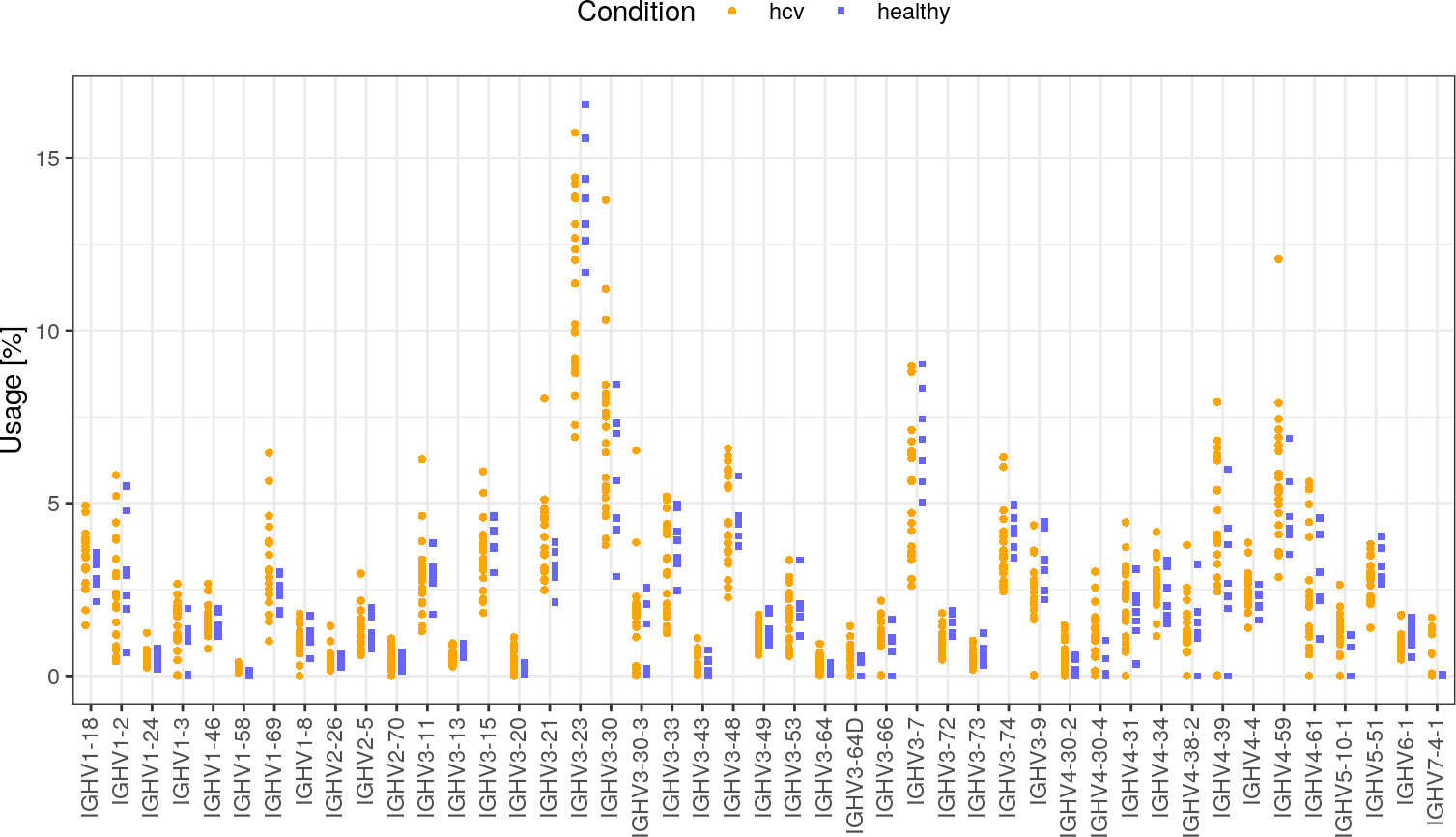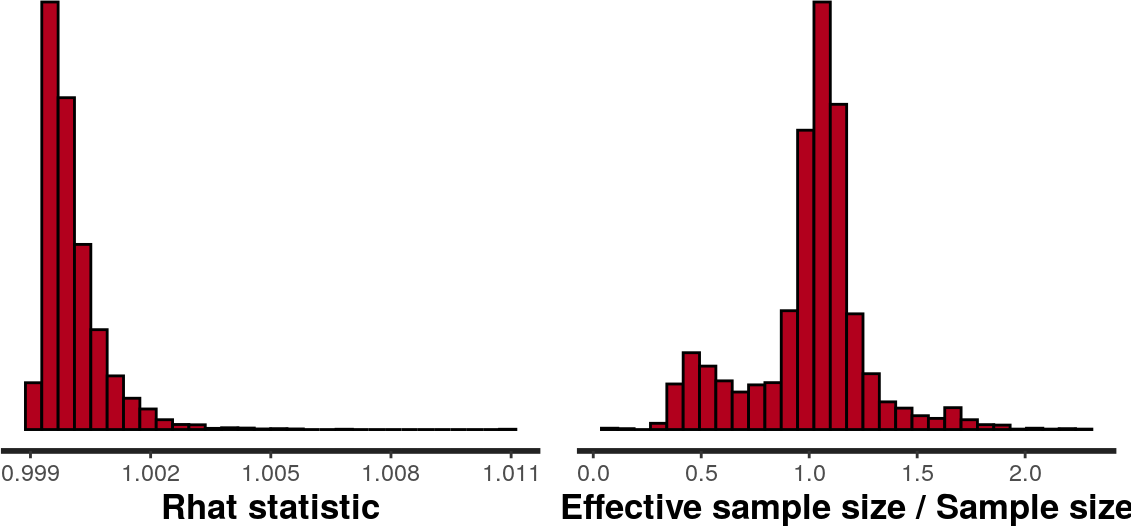# Contents

require(IgGeneUsage)
require(knitr)
require(ggplot2)
require(ggforce)
require(gridExtra)
require(ggrepel)
require(rstan)
require(reshape2)
rstan_options(auto_write = TRUE)

# 1 Introduction

Decoding the properties of immune repertoires is key in understanding the response of adaptive immunity to challenges such as viral infection. One important property is biases in Ig gene usage between biological conditions (e.g. healthy vs turmor). Yet, most analyses for differential gene usage are performed qualitatively, or with inadequate statistical methods. Here we introduce IgGeneUsage, a computational tool for the analysis of differential gene usage.

# 2 Input

The input to IgGeneUsage is a data.frame object with usage frequencies for each gene of a repertoire that belongs to a particular biological condition. The usage data.frame has the following 4 columns:

1. sample_id: identifier of the repertoire (e.g. Patient-1)
2. condition: identifier of the condition to which each repertoire belongs (e.g. healthy or tumor)
3. gene_name: specific gene name (e.g. IGHV1-10 or family TRVB1)
4. gene_usage_count: numeric (count) of usage related to columns 1-3

The sum of all gene usage counts (column 4) for a given repertoire should be equal to the total gene usage in that repertoire.

# 3 Method

IgGeneUsage transforms the provided input in the following way. Given $$R$$ repertoires, each having $$G$$ genes, IgGeneUsage generates a gene usage matrix $$Y^{R \times G}$$. Row sums in $$Y$$ define the total usage in each repertoire ($$N$$). The design variable $$X$$ is set to $$X = 1$$ for repertoires that belong to the first condition, and $$X = -1$$ otherwise.

For the analysis of DGU between two biological conditions, we designed the following Bayesian model ($$M$$) for zero-inflated beta-binomial regression. This model can fit over-dispersed gene usage data. The immune repertoire data is also not exhaustive, which leads to misdetection of genes that are systematically rearranged at low probability. The zero-inflated component of our model accounts for this:

\begin{align} p(Y_{ij} \mid M) = \begin{cases} \kappa + (1 - \kappa) \operatorname{BB}\left(0 \mid N_{i}, \theta_{ij}, \phi \right), & \text{if Y_{ij} = 0} \\ (1 - \kappa) \operatorname{BB}\left(Y_{ij} \mid N_{i}, \theta_{ij}, \phi \right), & \text{if Y_{ij} > 0} \end{cases}\\ \theta_{ij} = \operatorname{logit^{-1}}\left(\alpha_{j}+\beta_{ij}X_{i}\right)\\ \beta_{ij}\sim\operatorname{Normal}\left(\gamma_{j},\gamma_{\sigma} \right)\\ \gamma_{j}\sim\operatorname{Normal}\left(\hat{\gamma},\hat{\gamma}_{\sigma} \right) \\ \alpha_{j}\sim\operatorname{Normal}\left(\hat{\alpha},\hat{\alpha}_{\sigma} \right) \\ \hat{\gamma} \sim \operatorname{Normal}\left(0, 5\right) \\ \hat{\alpha} \sim \operatorname{Normal}\left(0, 10\right) \\ \gamma_{\sigma}, \hat{\gamma}_{\sigma}, \hat{\alpha}_{\sigma} \sim \operatorname{Cauchy^{+}}\left(0, 1\right) \\ \phi \sim \operatorname{Exponential}\left(\tau\right) \\ \tau \sim \operatorname{Gamma}\left(3, 0.1\right) \\ \kappa \sim \operatorname{Beta}\left(1, 3\right) \end{align}

Model $$M$$ legend:

• $$i$$ and $$j$$: index of different repertoires and genes, respectively
• $$\kappa$$: zero-inflation probability
• $$\theta$$: probability of gene usage
• $$\phi$$: dispersion
• $$\alpha$$: intercept/baseline gene usage
• $$\beta$$: slope/within-repertoire DGU coefficient
• $$\gamma$$, $$\gamma_{\sigma}$$: slope/gene-specific DGU coefficient; standard deviation
• $$\hat{\gamma}$$, $$\hat{\gamma}_{\sigma}$$: mean and standard deviation of the population of gene-specific DGU coefficients
• $$\hat{\alpha}$$, $$\hat{\alpha}_{\sigma}$$: mean and standard deviation of the population of gene-specific baseline usages
• $$\operatorname{BB}$$: beta-binomial probability mass function (pmf)
• $$\operatorname{Normal}$$: normal probability density function (pdf)
• $$\operatorname{Cauchy^{+}}$$: half-Cauchy pdf
• $$\operatorname{Exponential}$$: exponential pdf
• $$\operatorname{Gamma}$$: gamma pdf
• $$\operatorname{Beta}$$: beta pdf
• $$\operatorname{logit^{-1}}$$: inverse logistic function

In the output of IgGeneUsage, we report the mean effect size ($$\gamma$$) and its 95% highest density interval (HDI). Genes with $$\gamma \neq 0$$ (e.g. if 95% HDI of $$\gamma$$ excludes 0) are most likely to experience differential usage. Additionally, we report the probability of differential gene usage ($$\pi$$): \begin{align} \pi = 2 \cdot \max\left(\int_{\gamma = -\infty}^{0} p(\gamma)\mathrm{d}\gamma, \int_{\gamma = 0}^{\infty} p(\gamma)\mathrm{d}\gamma\right) - 1 \end{align} with $$\pi = 1$$ for genes with strong differential usage, and $$\pi = 0$$ for genes with negligible differential gene usage. Both metrics are computed based on the posterior distribution of $$\gamma$$, and are thus related. We find $$\pi$$ slightly easier to interpret.

# 4 Case Study I

IgGeneUsage provides built-in datasets from studies that evaluate biases in Ig gene usage. The dataset IGHV_HCV contains publicly available data of human immunoglobulin heavy chain VDJ rearrangements from a study that evaluates the effect of HCV infection on the human BCR repertoire1 Tucci, Felicia A., et al. “Biased IGH VDJ gene repertoire and clonal expansions in B cells of chronically hepatitis C virus–infected individuals.” Blood 131.5 (2018): 546-557.]. The dataset consists of a population of class-switched memory (CSM) B cells of 22 HCV-infected patients (HCV+) and 7 healthy donors (HD). Gene usage data is available for 69 IGHV gene segments.

## 4.1 Input data

The data is already formatted such that it can directly be passed as input to IgGeneUsage. The following steps allow you to load the data, and to inspect its column names and table entries.

data("IGHV_HCV", package = "IgGeneUsage")
kable(x = head(IGHV_HCV), row.names = FALSE)
sample_id condition gene_name gene_usage_count
HD1 healthy IGHV1-18 69
HD1 healthy IGHV4-30-2 16
HD1 healthy IGHV7-4-1 0
HD1 healthy IGHV3-19 1
HD1 healthy IGHV3-33 110
HD1 healthy IGHV3-69-1 4

## 4.2 Data visualization

Lets visualize the gene usage with ggplot2.

# we can compute the total number of rearrangements per sample
total.usage <- aggregate(gene_usage_count~sample_id+condition,
FUN = sum, data = IGHV_HCV)
total.usage$total <- total.usage$gene_usage_count
total.usage$gene_usage_count <- NULL # merge it with the original data viz <- merge(x = IGHV_HCV, y = total.usage, by = c("sample_id", "condition"), all.x = TRUE) # compute % viz$gene_usage_pct <- viz$gene_usage_count/viz$total*100

# For this example lets only consider the top 45 genes
# Hint: In real analyses you should not filter any gene
top <- aggregate(gene_usage_pct~gene_name, data = viz, FUN = mean)
top <- top[order(top$gene_usage_pct, decreasing = TRUE), ] IGHV_HCV <- IGHV_HCV[IGHV_HCV$gene_name %in% top$gene_name[seq_len(45)], ] viz <- viz[viz$gene_name %in% top$gene_name[seq_len(45)], ] # visualize ggplot(data = viz)+ geom_point(aes(x = gene_name, y = gene_usage_pct, fill = condition, shape = condition), position = position_dodge(width = .7), stroke = 0)+ theme_bw(base_size = 11)+ ylab(label = "Usage [%]")+ xlab(label = '')+ theme(legend.position = "top")+ theme(axis.text.x = element_text(angle = 90, hjust = 1, vjust = 0.4))+ scale_fill_manual(name = "Condition", values = c("orange", "#6666ff"))+ scale_shape_manual(name = "Condition", values = c(21, 22))## 4.3 Analysis of differential gene usage with IgGeneUsage This is the main method of IgGeneUsage. The primary input is the dataset IGHV_HCV. Other inputs allow you to configure specific settings of the Markov Chain Monte Carlo (MCMC) simulation. In this example we analyze IGHV_HCV with 2 MCMC chains (750 iterations each, including 250 warm-ups) in parallel using 2 CPU cores. We compute for each parameter of our model its 95% highest density interval (HDIs). Important remark: you should run your analysis using the default argument values of the function DGU. These values have been carefully chosen to fit most analyses for differential gene usage of immune repertoires. If any messages or warnings are reported concerning the MCMC sampling, please consult the Stan manual and adjust the MCMC arguments accordingly. If the warnings persist, please submit an issue with a reproducible script at the Bioconductor support site or on Github. M <- DGU(usage.data = IGHV_HCV, # input data mcmc.warmup = 750, # how many MCMC warm-ups per chain (default: 500) mcmc.steps = 2000, # how many MCMC steps per chain (default: 1,500) mcmc.chains = 2, # how many MCMC chain to run (default: 4) mcmc.cores = 1, # how many PC cores to use? (e.g. parallel chains) hdi.level = 0.95, # highest density interval level (de fault: 0.95) adapt.delta = 0.95, # MCMC target acceptance rate (default: 0.95) max.treedepth = 10) # tree depth evaluated at each step (default: 12) FALSE FALSE SAMPLING FOR MODEL 'zibb' NOW (CHAIN 1). FALSE Chain 1: FALSE Chain 1: Gradient evaluation took 0.001764 seconds FALSE Chain 1: 1000 transitions using 10 leapfrog steps per transition would take 17.64 seconds. FALSE Chain 1: Adjust your expectations accordingly! FALSE Chain 1: FALSE Chain 1: FALSE Chain 1: Iteration: 1 / 2000 [ 0%] (Warmup) FALSE Chain 1: Iteration: 250 / 2000 [ 12%] (Warmup) FALSE Chain 1: Iteration: 500 / 2000 [ 25%] (Warmup) FALSE Chain 1: Iteration: 750 / 2000 [ 37%] (Warmup) FALSE Chain 1: Iteration: 751 / 2000 [ 37%] (Sampling) FALSE Chain 1: Iteration: 1000 / 2000 [ 50%] (Sampling) FALSE Chain 1: Iteration: 1250 / 2000 [ 62%] (Sampling) FALSE Chain 1: Iteration: 1500 / 2000 [ 75%] (Sampling) FALSE Chain 1: Iteration: 1750 / 2000 [ 87%] (Sampling) FALSE Chain 1: Iteration: 2000 / 2000 [100%] (Sampling) FALSE Chain 1: FALSE Chain 1: Elapsed Time: 290.554 seconds (Warm-up) FALSE Chain 1: 341.985 seconds (Sampling) FALSE Chain 1: 632.539 seconds (Total) FALSE Chain 1: FALSE FALSE SAMPLING FOR MODEL 'zibb' NOW (CHAIN 2). FALSE Chain 2: FALSE Chain 2: Gradient evaluation took 0.001437 seconds FALSE Chain 2: 1000 transitions using 10 leapfrog steps per transition would take 14.37 seconds. FALSE Chain 2: Adjust your expectations accordingly! FALSE Chain 2: FALSE Chain 2: FALSE Chain 2: Iteration: 1 / 2000 [ 0%] (Warmup) FALSE Chain 2: Iteration: 250 / 2000 [ 12%] (Warmup) FALSE Chain 2: Iteration: 500 / 2000 [ 25%] (Warmup) FALSE Chain 2: Iteration: 750 / 2000 [ 37%] (Warmup) FALSE Chain 2: Iteration: 751 / 2000 [ 37%] (Sampling) FALSE Chain 2: Iteration: 1000 / 2000 [ 50%] (Sampling) FALSE Chain 2: Iteration: 1250 / 2000 [ 62%] (Sampling) FALSE Chain 2: Iteration: 1500 / 2000 [ 75%] (Sampling) FALSE Chain 2: Iteration: 1750 / 2000 [ 87%] (Sampling) FALSE Chain 2: Iteration: 2000 / 2000 [100%] (Sampling) FALSE Chain 2: FALSE Chain 2: Elapsed Time: 294.876 seconds (Warm-up) FALSE Chain 2: 336.868 seconds (Sampling) FALSE Chain 2: 631.743 seconds (Total) FALSE Chain 2: ## 4.4 Output The following objects are provided as part of the output of DGU: • glm.summary (main results of IgGeneUsage): quantitative summary of differential gene usage (see section ‘Results’) • test.summary: alternative quantitative summary of differential gene usage estimated with two frequentist methods (the Welch’s t-test and the Wilcoxon signed-rank test) • glm: rstan (‘stanfit’) object of the fitted model $$rightarrow$$ you can use this for further checks of your model (see section ‘Model checking’) • ppc.data: data on posterior predictive checks (see section ‘Model checking’) summary(M) FALSE Length Class Mode FALSE glm.summary 9 data.frame list FALSE test.summary 9 data.frame list FALSE glm 1 stanfit S4 FALSE ppc.data 2 -none- list FALSE usage.data 8 -none- list ## 4.5 Model checking Do not blindly trust your model $$\rightarrow$$ check it extensively. You can use the object glm for these checks. • Checklist of successful MCMC sampling: • no divergences, no excessive warnings from rstan, Rhat < 1.05, high Neff • Checklist for valid model: • observed data can be replicated with the fitted model $$\rightarrow$$ posterior predictive checks • leave-one-out analysis ### 4.5.1 MCMC sampling • divergences, tree-depth, energy rstan::check_hmc_diagnostics(M$glm)
FALSE
FALSE Divergences:
FALSE
FALSE Tree depth:
FALSE
FALSE Energy:
• Rhat and Neff
gridExtra::grid.arrange(rstan::stan_rhat(object = M$glm), rstan::stan_ess(object = M$glm),
nrow = 1)### 4.5.2 Posterior predictive checks: repertoire-specific

IgGeneUsage has built-in checks for repertoire-specific posterior prediction of gene usage. It uses the fitted model to predict the usage of each gene in a given repertoire (y-axis). Error bars show 95% HDI of mean posterior prediction. The predictions can be compared with the observed data (x-axis). For points near the diagnoal $$\rightarrow$$ accurate prediction.

The following figure shows that our model can reproduce the fitted data $$\rightarrow$$ this is an indicator of a valid model.

ggplot(data = M$ppc.data$ppc.repertoire)+
facet_wrap(facets = ~sample_name, ncol = 5)+
geom_abline(intercept = 0, slope = 1, linetype = "dashed", col = "darkgray")+
geom_errorbar(aes(x = observed_count, y = ppc_mean_count,
ymin = ppc_L_count, ymax = ppc_H_count), col = "darkgray")+
geom_point(aes(x = observed_count, y = ppc_mean_count,
fill = condition), shape = 21, size = 1)+
theme_bw(base_size = 11)+
theme(legend.position = "top")+
scale_x_log10(breaks = c(1, 10, 100, 1000),
labels = expression(10^0, 10^1, 10^2, 10^3))+
scale_y_log10(breaks = c(1, 10, 100, 1000),
labels = expression(10^0, 10^1, 10^2, 10^3))+
xlab(label = "Observed usage [counts]")+
ylab(label = "Predicted usage [counts]")+
annotation_logticks(base = 10, sides = "lb")